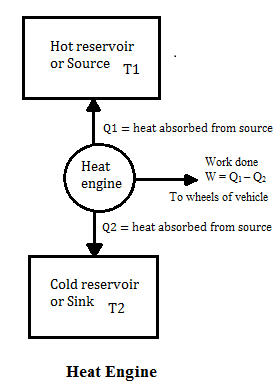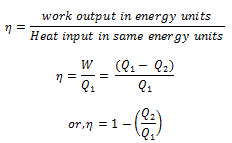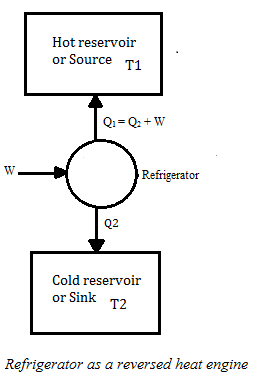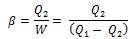# Heat Engine and efficiency

A heat engine is a device by which a system is made to undergo a cyclic process that results in the conversion of heat to work.

For any heat engine, there are three essential requirements.

1. SOURCE: A hot body at fixed temperature T1 from which heat engine can draw heat
2. SINK: A cold body, at a fixed lower temperature T2, to which any amount of heat can be rejected.
3. WORKING SUBSTANCE: The material, which on being supplied with heat will do mechanical work.

## Working Substance:

• In the heat engine, working substances could be gas in a cylinder with a moving piston.
• In the heat engine, working substance takes heat from the source, converts a part of it into mechanical work, gives out rest to the sink and returns to the initial state. This series of operations constitutes a cycle.
• This cycle is represented in fig below• Work from heat engine can be continuously obtained by performing same cycle again and again.
• Consider,

Q1 = heat absorbed by working substance from source

Q2 = heat rejected to the sense

W = net amount of work done by working substance

Q1 – Q2 = net amount of heat absorbed by working substance

∆U = 0 since in the cycle Working substance returns to its initial condition.

So on application of first law of thermodynamics

Q1 – Q2 = W

• ### Thermal efficiency of heat engineFrom this equation it is clear that

Q = 1 for Q2 = 0

And there would be 100% conversion of heat absorbed into work but such ideal engines are not possible in practice.

# Refrigerators and Heat Pumps

1. Refrigerators work in reverse direction of heat engines.
2. In refrigerators working substance extracts heat Q2 from sink at lower temperature T2
3. Some external work is performed by the compressor of the refrigerator and then heat Q1 is rejected to the source, to the radiator of the refrigerator.## The coefficient of performance:

β= Amount of heat absorbed from the cold reservoir/work done in running the machinery
Q2 – heat absorbed from cold reservoir.
Q1 – heat rejected to hot reservoir during one complete cycle
W = (Q1-Q2) is the work done in running the machinery

thus,1. Like heat engines, refrigerators cannot work without some external work done on the system. Hence coefficient of performance cannot be infinite.